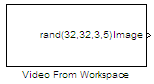# Video From Workspace

Import video from MATLAB workspace

• Library:
• Computer Vision Toolbox / Sources

•## Description

The Video From Workspace block imports a video from the MATLAB® workspace. The output of the block depends on the format of the video in the workspace.

• If the video is represented by an M-by-N-by-T array, the block outputs a grayscale video. M and N are the number of rows and columns in each video frame, and T is the number of frames in the video.

• If the video is represented by an M-by-N-by-C-by-T array, the block outputs a color video. M and N are the number of rows and columns in each video frame, C is the number of color channels, and T is the number of frames in the video.

Note

• If you generate code from a model that contains this block, it takes long to compile the code as the Simulink® Coder™ puts all of the video data into the `.c` file. To shorten code generation time, convert your video data into a format supported by the From Multimedia File or Read Binary File block.

• The Video From Workspace block also supports `fi` objects. For more information on `fi` objects, see fi Object Properties (Fixed-Point Designer).

## Ports

### Output

expand all

Output image or video, returned as an M-by-N-by-T array or M-by-N-by-C-by-T array. If the output is a 3-D array, the third dimension is the number of frames in the video. If the output is a 4-D array, the third dimension is the number of color channels, and the fourth dimension is the number of frames in the video.

Note

If an RGB image of size, M-by-N-by-3 array is imported from the MATLAB workspace, the block considers the third dimension to be the number of frames and processes the image as a grayscale video with 3 identical frames.

Data Types: `single` | `double` | `int8` | `int16` | `int32` | `uint8` | `uint16` | `uint32` | `Boolean` | `fixed point`

Red color component of the input video, returned as a 3-D array. The third dimension is the number of frames in the input video.

Note

The port label can be changed by using the Output port labels parameter.

#### Dependencies

To enable this port, set the Image signal parameter to `Separate color signals`.

Data Types: `single` | `double` | `int8` | `int16` | `int32` | `uint8` | `uint16` | `uint32` | `Boolean` | `fixed point`

Green color component of the input video, returned as a 3-D array. The third dimension is the number of frames in the input video.

Note

The port label can be changed by using the Output port labels parameter.

#### Dependencies

To enable this port, set the Image signal parameter to `Separate color signals`.

Data Types: `single` | `double` | `int8` | `int16` | `int32` | `uint8` | `uint16` | `uint32` | `Boolean` | `fixed point`

Blue color component of the input video, returned as a 3-D array. The third dimension is the number of frames in the input video.

Note

The port label can be changed by using the Output port labels parameter.

#### Dependencies

To enable this port, set the Image signal parameter to `Separate color signals`.

Data Types: `single` | `double` | `int8` | `int16` | `int32` | `uint8` | `uint16` | `uint32` | `Boolean` | `fixed point`

## Parameters

expand all

Specify the video to read from the workspace. The value for this parameter can be a 3-D numeric array, 4-D numeric array, or a MATLAB workspace variable.

To import an AVI file from the MATLAB workspace, use this process.

1. Read the file by using the `VideoReader` function.

```mov = VideoReader('filename.avi') ```
2. Specify the workspace variable, in this case mov as the value for the Signal parameter. If the AVI file has an associated colormap, it must satisfy these conditions.

• The colormap must be empty or have 256 values.

• The data must represent a grayscale image.

• The data type of the pixel values must be `uint8`.

This parameter specifies the sample period of the output video as a positive scalar or `-1`. When set to `-1`, the block inherits the sample time from the simulink model.

Specify the block output for the duration of the simulation after the block generates the final video frame.

• `Setting to zero` — Generate zero-valued outputs.

• `Holding final value` — Repeat the final frame.

• `Cyclic repetition` — Repeat the video from the beginning.

Specify the format in which the block returns a color video.

• `One multidimensional signal` — Return all color channels of the video in a single 4-D array. The third dimension is the number of color channels in the video.

• `Separate color signals` — Return each color channel of the video as a separate 3-D array. This setting enables a number of output ports on the block equal to the number of color channels in the video. Each port returns a single color channel.

Name the output ports. Use the vertical bar character, |, as a delimiter.

Example:: Red|Green|Blue

#### Dependencies

To enable this parameter, set the Image signal parameter to `Separate color signals`.

## Block Characteristics

 Data Types `Boolean` | `double` | `fixed point` | `integer` | `single` Multidimensional Signals `yes` Variable-Size Signals `no`

## Version History

Introduced before R2006a